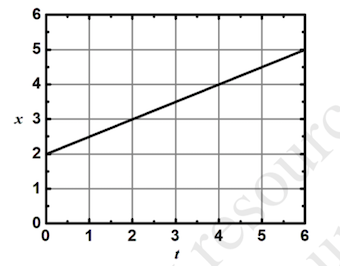# Problem: For the curve in the following figure, find x as a function of t.

###### FREE Expert Solution
91% (118 ratings)
###### Problem Details

For the curve in the following figure, find x as a function of t.Frequently Asked Questions

What scientific concept do you need to know in order to solve this problem?

Our tutors have indicated that to solve this problem you will need to apply the Graphing Position, Velocity, and Acceleration Graphs concept. You can view video lessons to learn Graphing Position, Velocity, and Acceleration Graphs. Or if you need more Graphing Position, Velocity, and Acceleration Graphs practice, you can also practice Graphing Position, Velocity, and Acceleration Graphs practice problems.

How long does this problem take to solve?

Our expert Physics tutor, Jeffery took 1 minute and 26 seconds to solve this problem. You can follow their steps in the video explanation above.